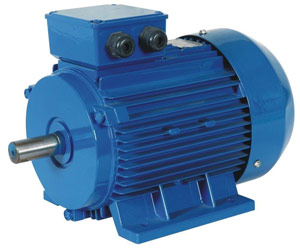Categories

# What is vector control of induction motor?

The stator voltage and current to an induction motor produces a magnetizing flux to transfer the stator energy to the rotor. In Scalar or V/Hz control, this control does not provide very accurate speed regulation typically in the 2-4% range. This accuracy is good enough for controlling fans and pumps, since the control parameter is not speed accuracy, but temperature or pressure control over an average time period. Also using scalar or V/Hz control, you cannot control zero speed, ie no holding torque capability.When we are looking at Vector control, rotor speed is the control parameter. The control scheme for Vector Control of an induction motor was written over 60 years ago, but the microprocessor was not invented with enough computing power to do the high degree of math calculations every microsecond to use this scheme until the mid-1980s. The first couple of years using Vector versions were not very accurate and the performance gain was compromised. The newer microprocessors of the late 1980s increased the computing speed and accuracy allowing the true Vector control performance to be a valid product.

The term Vector control uses the instantaneous position and magnitude of the Vectors of the Rotor and Stator Voltages and Currents to determine the rotor position with respect to the stator field. In most cases, auto tuning must be accomplished to determine the Vector positions for small signal step changes and for larger signal step changes. The microprocessor learns the angle differences and now provides speed accuracy to +/- 0.001 RPM over the speed range.

There are two unfortunate issues with no encoder feedback:

1. The Current and Voltage vectors are temperature dependent, meaning that if the tuning was done at room temperature motor and not at normal load the induction motor is 80 degrees hotter, then the vector calculation goes out of accuracy, therefore induction motor temperature feedback is required. (Note 80 degrees C change will have a significant increase in the resistance portion of the Vectors circuits.)
2. The Voltage or Current Vector math algorithm calculations do not provide required accuracy is over the entire speed range. The Voltage model has extremely high accuracy the faster the speed, however once you try to control the rotor speed below 20RPM, the error increases rapidly. The Current Method has consistent accuracy over the entire speed range from zero to maximum speed. In the 0 -3 Hertz range the current method of doing the calculations is more accurate than the voltage method. Many Manufactures today have chosen to use both Current and Voltage control methods. They would program in the Current calculation control for 0 to 5 Hertz and switch to Voltage calculations above 5 Hertz. A 1 to 1.5 Hertz bandwidth will be used to prevent switching back and forth between the two control methods.

The Vector controller now sends two independent and controllable signals to the induction motor:

1. A current signal ~90 degrees out of phase with the stator voltage to produce the rated flux current between the rotor and stator. Once this field signal is applied, the rotor and stator are locked together.
2. An instantaneous frequency signal to the stator to produce a rotating field around the rotor, causing the rotor to follow the stator frequency. If the instantaneous Voltage and current vectors are not as calculated, then the flux is instantaneously changed to correct for the error. The performance is zero speed torque with excellent speed control.

Using and encoder that provides say 5000 pulses per revolution, means we can control the rotor position to 1/5000 of an RPM from the stator field. The encoder now provides the speed accuracy of the induction motor over the entire speed range. The requirement for knowing the induction motor temperature or the instantaneous values of the Voltage and Current Vectors becomes muted.